Next: Ocean Sciences Building Amenities. Up: PTV Operating Guidelines Previous: Compressibility of the PTV.   Contents

Calculation of Potential Energy Stored in Compressed Water.

Consider a cylinder capped on one end with an end cap and on the other end with a piston (see Fig. 3). The cylinder is filled with a mass,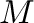, of water which is pressurized by applying a force,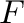, to the piston. The force that must be applied is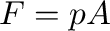where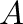is the area of the piston and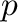is the water pressure. The work done in compressing the water is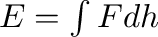,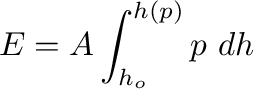(9)

where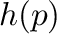and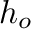are the piston positions at pressureand at atmospheric pressure, respectively. This quantity of energy is stored in the water as potential energy and represents the maximum that might hypothetically be converted to kinetic energy during vessel failure.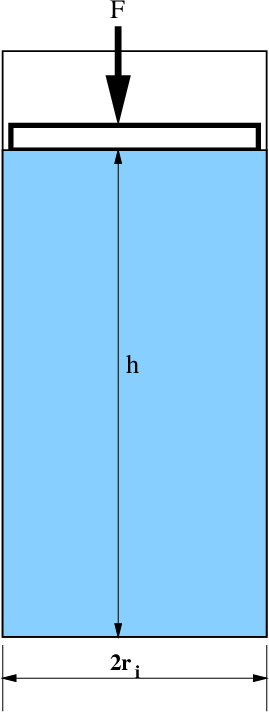The fact that the mass of water is fixed can be used to determine the relationship between the piston position and the water pressure. Note that a fixed mass implies that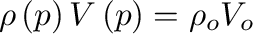(10)

where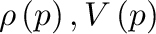are the water density and volume of the water at pressure,. The quantities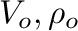are measured at atmospheric pressure. The dependence of water density on pressure can be expressed as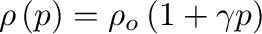(11)

where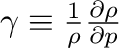is the compressibility of water. Table 3 demonstrates that the compressibility is nearly constant over the range of pressures relevant to the PTV.

Table 3: The compressibility of water at a temperature of 20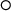C. The compressibility varies by less than 20% over the range of pressures and temperatures that are applicable to the PTV.
 Pressure (psi) Compressibility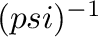0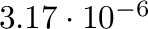2500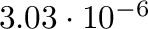5000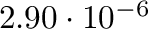7500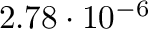10000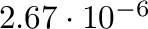The density can also be expressed as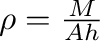whereis the cross-sectional-area of the vessel. Substituting this into (11) yields the following equivalent relationships between the pressure and the piston position,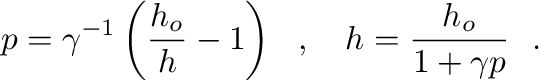(12)

Substituting (12) into (9) yields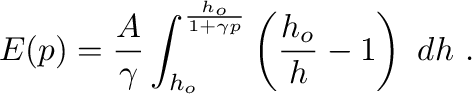(13)

Evaluating this integral gives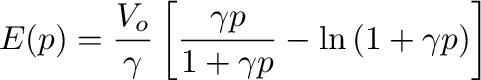(14)

Equation (14) is exact but unnecessarily complicated. Note that even at a maximum pressure of 10 kpsi then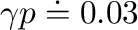and therefore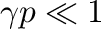. Computing the Taylor series of (14) and neglecting third and higher order terms yields a greatly simplified expression with an error of approximately only 3%: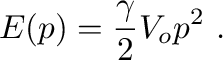(15)Next: Ocean Sciences Building Amenities. Up: PTV Operating Guidelines Previous: Compressibility of the PTV.   Contents
Dana Swift, swift@ocean.washington.edu